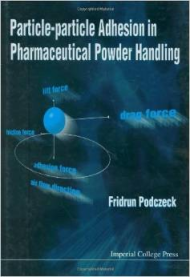### Quick Start

The way particles stick to each other and to surfaces is tricky. Three apps on this page cover Particle-Surface, Particle Clumping, Particle Engulfment. If any of these issues is of interest to you then you'll find that the science is straightforward and the apps are simple to use.

The ways that particles stick to themselves and to surfaces are important for everything to do with our ability to handle them in a controlled manner. This page discusses the basics - which rely on the familiar JKR concepts already covered.The best resource I know for understanding particle adhesion is the book by Prof Fridrun Podczeck, Particle-Particle Adhesion in Pharmaceutical Powder Handling, World Scientific Publishing, 1998, from which I have taken most of the equations in what follows. Prof Podczeck is herself a source of much of the high-grade academic data on the subject. The book extensively accesses the older resource of Zimon's Adhesion of Dust and Powder.

From basic JKR theory for a sphere of radius R and for a work of adhesion W between the sphere and the surface, the force needed to remove the particle is

F_(adh)=1.5πRW.

It is instructive to compare this (weak) force with that of gravity. Given a particle density of ρ, the gravitational force is F_g= 4/3πR^3ρg, and the ratio F_(adh)/F_g is shown. For small particles (<1mm) this is much greater than 1, meaning that gravity is insignificant in dislodging small particles.

If water vapour is present and the RH>50% then a liquid meniscus spontaneously forms between the surface and the particle. The result is a capillary force, F_c=4πRγ_Lcos(θ) + 4πRγ_(SL). The app shows that the capillary forces are much larger than the adhesion forces, so for free flow of powders it is vital to keep RH<50%. However, at even higher %RH water can start to condense around the particle and getting between it and the surface. This can greatly decrease the resulting adhesion. Finally, the water might start a "sintering" process by creating bridges between particles via solution/dissolution. In other words, the effects of RH are complex but the basic message is the same: try to keep below 50% if you want the powder to flow freely.

W mJ/m2
Liquid γL mN/m
θ °
Surface γSL mN/m
Density ρ g/cc
Capillary Fc N

The above is highly idealistic - assuming smooth particles and a smooth surface. The effect of roughness is, initially, to greatly decrease adhesion because there is less direct contact. But larger roughnesses can increase adhesion if the particles can get trapped within valleys. The effect can be even stronger if the valleys are grooves and the particles are cylinders. In other words, there is no simple way to think through the effects of roughness.

However, lower-modulus particles can conform better to a rough surface so there should be some sort of formula relating adhesion to radius, roughness and modulus. The Fuller-Tabor formula does that by calculating an adhesion parameter (AP) where the higher the value the lower the adhesion, with values > 10 being effectively zero adhesion. For a particle of modulus E (actually reduced modulus, corrected for Poisson, taken as 0.33), Work of Adhesion W, Radius R and an RMS roughness σ (Rq from a stylus probe):

AP=(Eσ)/W sqrt(σ/R).

Finally, for reasons ultimately related to JKR-style thinking, particles of radius R will tend to spontaneously clump (during typical types of handling) into particles of diameter D given by:

D=0.064[(2E(2R)^(5/2))/W]^(2/3)

W mJ/m2
Modulus E MPa
Roughness σ μm